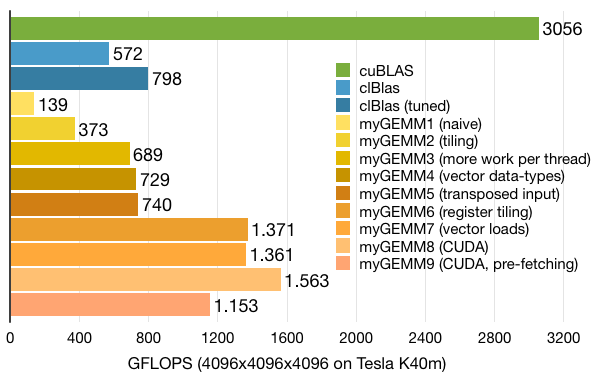# Tutorial: OpenCL SGEMM tuning for Kepler

Note: the complete source-code is available at GitHub.
Note2: a tuned OpenCL BLAS library based on this tutorial is now available at GitHub.
Note3: a WebGL2 demo of this tutorial is available at: https://www.ibiblio.org/e-notes/webgl/gpu/mul/sgemm.htm.

## Kernel 9: Pre-fetching

Still not at the performance level of cuBLAS, so what's next? Are we using the same techniques as cuBLAS? Inspection using the CUDA visual profiler tells us that cuBLAS is running only a single work-group of 256 threads per SM, that each work-group uses 32KB local memory, and that each thread consumes the maximum of 255 registers. Let's try to mimic that configuration.

First of all, if we go for only 256 threads per SM, we'll suffer from the latency of off-chip memory accesses. There is a well-known technique to overcome that: software pre-fetching. In the case of matrix-multiplication, that means that we are going to load the next tile while we are computing the current tile, such that the latency of the loads can be hidden by computations.

To implement this, we'll need twice as much local memory, automatically limiting us to one work-group per SM (and giving us the same 32KB as cuBLAS). Since we cannot have 3D arrays, we'll just flatten one dimension:

```// Local memory to fit two tiles of A and B
__local float Asub[TSK*TSM];
__local float Bsub[TSK*TSN];
```

Since the kernel code has become quite large, we'll take a look at a shorter pseudo-code version. The code below replaces the previous loop over the tiles. Things to notice:

• Before starting the loop, we already load the first tile (tile 0) into Asub[0,*] and Bsub[0,*]. After that, we have to synchronise because there is no other barrier before the start of the computation.
• Within the loop we already load the next tile. Note that we need an extra if-statement for when we arrive at the last tile.
• The computation is as before, but now alternates between the two tiles of Asub and Bsub. With pre-fetching, we no longer need to synchronise directly before computation, allowing overlap between loads and FMAs.

```// Load the first tile of A and B into local memory

// Loop over all tiles
int numTiles = K/TSK;
for (int t=0; t<numTiles; t++) {

// Synchronise
barrier(CLK_LOCAL_MEM_FENCE);

// Load the next tile of A and B into local memory
int tt = t + 1;
if (tt<numTiles) {
}

// Perform the computation
compute(tile=t, Asub[t%2][*], Bsub[t%2][*]);
}
```

Since pre-fetching uses twice as much local memory, we end up with only 256 active threads. Although we do pre-fetch, this still harms performance significantly, ending up with ~400 GFLOPS less than the previous kernel. However, we no longer have to care about the 128 registers per thread limit to get 512 threads running, so we have headroom for further optimisations. On to the next kernel!Tutorial written by Cedric Nugteren, (c) 2014 SURFsara
Last updated in 2014 - information might be outdated